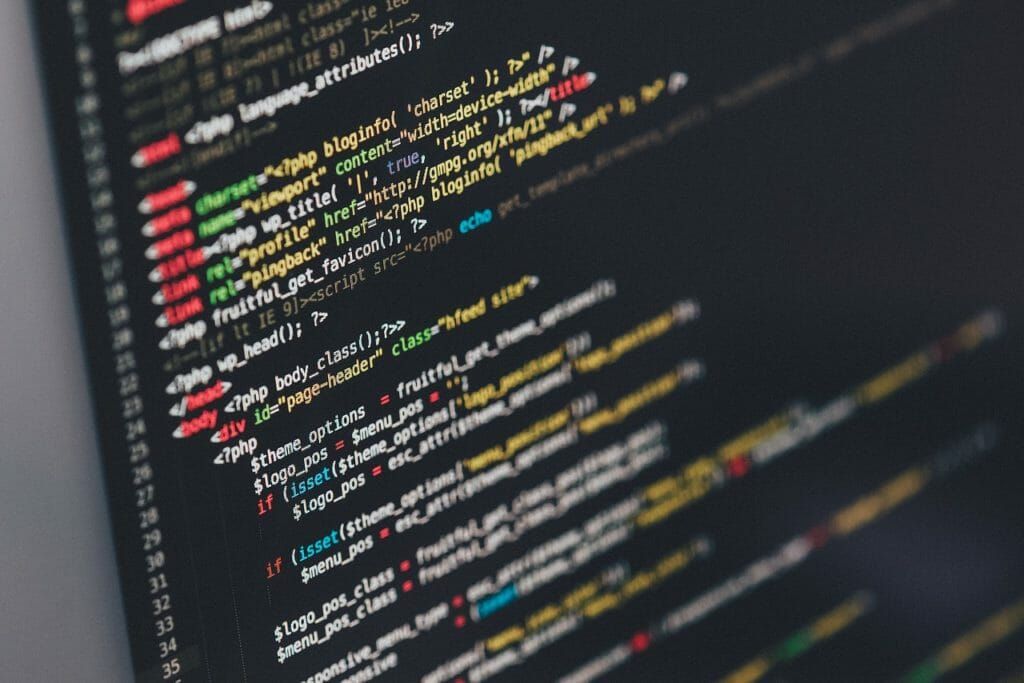# [Python] Update column value of Pandas DataFrameThere are some ways to update column value of Pandas DataFrame.
So I wrote down them for the future reference.
I used documents of `loc` and `where` below as a reference.

## Update column value of Pandas DataFrame

There are some ways to update column value of Pandas DataFrame.
Common ways are below.

• Bulk update by single value
• Update rows that match condition
• Update with another DataFrame

I'll introduce them with using DataFrame sample.

```import pandas as pd

data_list1 = [
[1,2,3],
[2,3,4],
[3,4,5]
]
col_list1 = ["c1","c2","c3"]
df1 = pd.DataFrame(data=data_list1, columns=col_list1)
print(df1)

#    c1  c2  c3
# 0   1   2   3
# 1   2   3   4
# 2   3   4   5
```

### Bulk update by single value

Bulk update is easy.
But it updates all rows. So it is not so useful and we can use it only for initialization.

```df1["c3"]=1
print(df1)
#    c1  c2  c3
# 0   1   2   1
# 1   2   3   1
# 2   3   4   1
```

### Update rows that match condition

If you want to update specific rows that match condition, you can use `loc`.

```df1.loc[df1["c3"]>3, ["c3"]]=1
print(df1)
#    c1  c2  c3
# 0   1   2   3
# 1   2   3   1
# 2   3   4   1
```

Without using `loc`, you will see warning and you will not update values.

```df1[df1["c3"]>3]["c3"]=1
print(df1)
# SettingWithCopyWarning:
# A value is trying to be set on a copy of a slice from a DataFrame.
# Try using .loc[row_indexer,col_indexer] = value instead
#    c1  c2  c3
# 0   1   2   3
# 1   2   3   4
# 2   3   4   5
```

As an another method, we can update unmatched rows with using `where`.
In the example below, it updates column "c3" values of rows that have less values than 4 in column "c2".

```df1["c3"].where(df1["c2"] >= 4, 10, inplace=True)  # Update unmatched cells
print(df1)

#    c1  c2    c3
# 0   1   2  10.0
# 1   2   3  10.0
# 2   3   4   1.0
```

### Update with another DataFrame

There is a way to update column with another DataFrame.
With using this method, we can choose certail rows from parent DataFrame and apply updated values to parent DataFrame after the child process.

```data_list2 = [
[5,7],
[6,8],
]
col_list2 = ["c4","c3"]
df2 = pd.DataFrame(data=data_list2, columns=col_list2)
print(df2)
#    c4  c3
# 0   5   7
# 1   6   8

df1.update(df2)
print(df1)
#    c1  c2   c3
# 0   1   2  7.0
# 1   2   3  8.0
# 2   3   4  1.0
```

コチラもオススメKRSW

Translate »